# Cartoon+Texture Variational Image Decomposition¶

Important: Please read the installation page for details about how to install the toolboxes. $\newcommand{\dotp}{\langle #1, #2 \rangle}$ $\newcommand{\enscond}{\lbrace #1, #2 \rbrace}$ $\newcommand{\pd}{ \frac{ \partial #1}{\partial #2} }$ $\newcommand{\umin}{\underset{#1}{\min}\;}$ $\newcommand{\umax}{\underset{#1}{\max}\;}$ $\newcommand{\umin}{\underset{#1}{\min}\;}$ $\newcommand{\uargmin}{\underset{#1}{argmin}\;}$ $\newcommand{\norm}{\|#1\|}$ $\newcommand{\abs}{\left|#1\right|}$ $\newcommand{\choice}{ \left\{ \begin{array}{l} #1 \end{array} \right. }$ $\newcommand{\pa}{\left(#1\right)}$ $\newcommand{\diag}{{diag}\left( #1 \right)}$ $\newcommand{\qandq}{\quad\text{and}\quad}$ $\newcommand{\qwhereq}{\quad\text{where}\quad}$ $\newcommand{\qifq}{ \quad \text{if} \quad }$ $\newcommand{\qarrq}{ \quad \Longrightarrow \quad }$ $\newcommand{\ZZ}{\mathbb{Z}}$ $\newcommand{\CC}{\mathbb{C}}$ $\newcommand{\RR}{\mathbb{R}}$ $\newcommand{\EE}{\mathbb{E}}$ $\newcommand{\Zz}{\mathcal{Z}}$ $\newcommand{\Ww}{\mathcal{W}}$ $\newcommand{\Vv}{\mathcal{V}}$ $\newcommand{\Nn}{\mathcal{N}}$ $\newcommand{\NN}{\mathcal{N}}$ $\newcommand{\Hh}{\mathcal{H}}$ $\newcommand{\Bb}{\mathcal{B}}$ $\newcommand{\Ee}{\mathcal{E}}$ $\newcommand{\Cc}{\mathcal{C}}$ $\newcommand{\Gg}{\mathcal{G}}$ $\newcommand{\Ss}{\mathcal{S}}$ $\newcommand{\Pp}{\mathcal{P}}$ $\newcommand{\Ff}{\mathcal{F}}$ $\newcommand{\Xx}{\mathcal{X}}$ $\newcommand{\Mm}{\mathcal{M}}$ $\newcommand{\Ii}{\mathcal{I}}$ $\newcommand{\Dd}{\mathcal{D}}$ $\newcommand{\Ll}{\mathcal{L}}$ $\newcommand{\Tt}{\mathcal{T}}$ $\newcommand{\si}{\sigma}$ $\newcommand{\al}{\alpha}$ $\newcommand{\la}{\lambda}$ $\newcommand{\ga}{\gamma}$ $\newcommand{\Ga}{\Gamma}$ $\newcommand{\La}{\Lambda}$ $\newcommand{\si}{\sigma}$ $\newcommand{\Si}{\Sigma}$ $\newcommand{\be}{\beta}$ $\newcommand{\de}{\delta}$ $\newcommand{\De}{\Delta}$ $\newcommand{\phi}{\varphi}$ $\newcommand{\th}{\theta}$ $\newcommand{\om}{\omega}$ $\newcommand{\Om}{\Omega}$

This numerical tour explores the use of variational energies to decompose an image into a cartoon and a texture layer.

In :
addpath('toolbox_signal')


A variational image separation finds a decomposition $f = u^\star + v^\star + w^\star$ where $u^\star$ and $v^\star$ are solutions of an optimization problem of the form $$\min_{u,v} \: \frac{1}{2}\|f-u-v\|^2 + \lambda J(u) + \mu T(v),$$

where $J(u)$ is a cartoon image prior (that favors edges) and $T(v)$ is a texture image prior (that favors oscillations). The parameters $\lambda,\mu$ should be adapted to the noise level and the amount of edge/textures.

When no noise is present in $f$, so that $w^\star=0$, on minimizes $$\min_{u} \: T(f-u) + \lambda J(u).$$

In this tour, we define $J$ as the total variation prior. For $T$, we use the Hilbert norm framework introduced in:

Constrained and SNR-based Solutions for TV-Hilbert Space Image Denoising, Jean-Fran ois Aujol and Guy Gilboa, Journal of Mathematical Imaging and Vision, volume 26, numbers 1-2, pages 217-237, November 2006.

## Total Variation Cartoon Prior¶

The total variation is a Banach norm. On the contrary to the Sobolev norm, it is able to take into account step edges.

First we load a textured image.

In :
n = 256;
name = 'boat';


Display it.

In :
clf;
imageplot(f);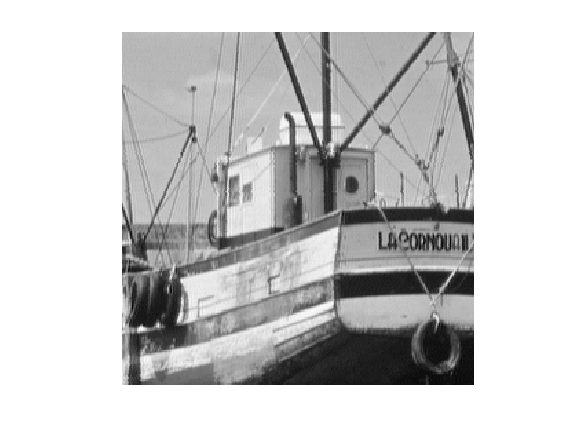The total variation of a smooth image $f$ is defined as $$J(f)=\int \|\nabla f(x)\| d x$$

It is extended to non-smooth images having step discontinuities.

The total variation of an image is also equal to the total length of its level sets. $$J(f)=\int_{-\infty}^{+\infty} L( S_t(f) ) dt$$

Where $S_t(f)$ is the level set at $t$ of the image $f$ $$S_t(f)=\{ x \backslash f(x)=t \}$$

The Gradient of the TV norm is $$\text{Grad}J(f) = \text{div}\left( \frac{\nabla f}{\|\nabla f\|} \right) .$$

The gradient of the TV norm is not defined if at a pixel $x$ one has $\nabla f(x)=0$. This means that the TV norm is difficult to minimize, and its gradient flow is not well defined.

To define a gradient flow, we consider instead a smooth TV norm $$J_\epsilon(f) = \int \sqrt{ \varepsilon^2 + \| \nabla f(x) \|^2 } d x$$

This corresponds to replacing $\|u\|$ by $\sqrt{\varepsilon^2 + \|u\|^2}$ which is a smooth function.

We display (in 1D) the smoothing of the absolute value.

In :
u = linspace(-5,5)';
clf;
subplot(2,1,1); hold('on');
plot(u, abs(u), 'b');
plot(u, sqrt(.5^2+u.^2), 'r');
title('\epsilon=1/2'); axis('square');
subplot(2,1,2); hold('on');
plot(u, abs(u), 'b');
plot(u, sqrt(1^2+u.^2), 'r');
title('\epsilon=1'); axis('square');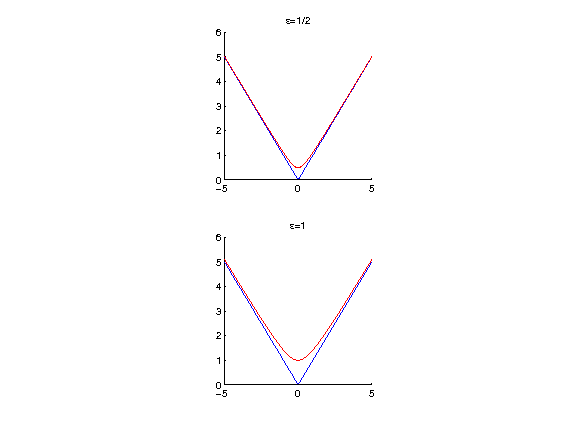In the following we set a small enough regularization parameter $\varepsilon$.

In :
epsilon = 1e-2;


Compute the (smoothed) total variation of $f$.

In :
J = @(u)sum(sum( sqrt( epsilon^2 + sum3(grad(u).^2,3) ) ));
disp(['J(f) = ' num2str(J(f),3)]);

J(f) = 4.37e+03


## TV-$L^2$ Model¶

The simplest decomposition method performs a total variation denoising: $$\min_u \frac{1}{2}\|f-u\|^2 + \lambda J(u).$$

It corresponds to the TV-$L^2$ model of Rudin-Osher-Fatermi, because the texture prior is the $L^2$ norm: $$T(v) = \frac{1}{2} \|v\|^2.$$

This a poor texture prior because it just assumes the texture has a small overall energy, and does not care about the oscillations.

Define the regularization parameter $\lambda$.

In :
lambda = .2;


The step size for diffusion should satisfy: $$\tau < \frac{2}{1 + \lambda 8 / \varepsilon} .$$

In :
tau = 1.9 / ( 1 + lambda * 8 / epsilon);


Initialization of the minimization.

In :
u = f;


The Gradient of the smoothed TV norm is $$\text{Grad}J(f) = \text{div}\left( \frac{\nabla f}{\sqrt{\varepsilon^2 + \|\nabla f\|^2}} \right) .$$

Shortcut for the gradient of the smoothed TV norm.

In :
GradJ0 = @(Gr)-div( Gr ./ repmat( sqrt( epsilon^2 + sum3(Gr.^2,3) ) , [1 1 2]) );


One step of descent.

In :
u = u - tau*( u - f + lambda* GradJ(u) );


Exercise 1

Compute the gradient descent and monitor the minimized energy.

In :
exo1()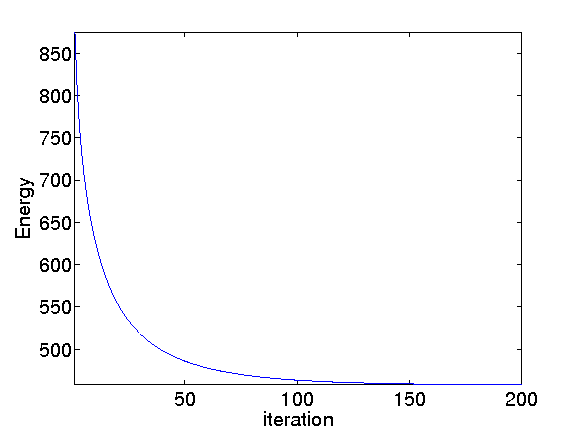In :
%% Insert your code here.


Display the cartoon layer.

In :
clf;
imageplot(u);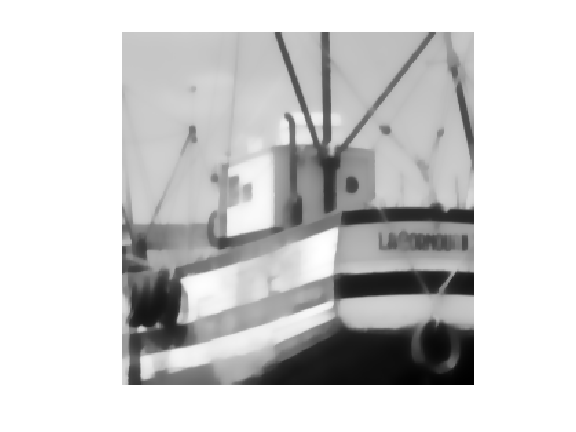Shortcut to increase the contrast of the textured layer for better display.

In :
rho = .8; % constrast factor
eta = .2; % saturation limit
displaytexture0 = @(x)sign(x).*abs(x).^rho;
displaytexture  = @(v)displaytexture0( clamp(v,-eta,eta)/eta );


Display the textured layer.

In :
clf;
imageplot( displaytexture(f-u) );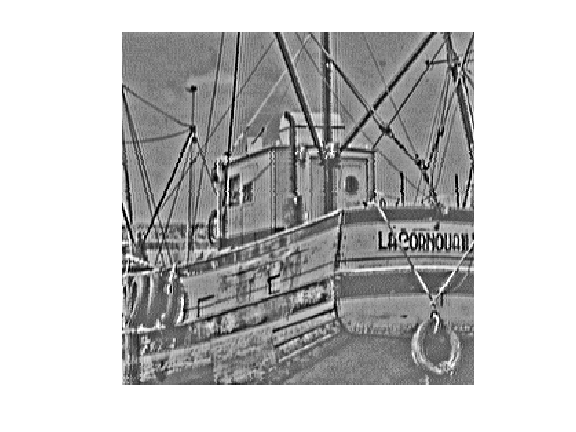## Gabor Hilbert Energy¶

To model the texture, one should use a prior $T(v)$ that favors oscillations. We thus use a weighted $L^2$ norms computed over the Fourier domain: $$T(v) = \frac{1}{2} \sum_{\omega} \|W_{\omega} \hat f(\omega) \|^2$$ where $W_\omega$ is the weight associated to the frequency $\omega$.

This texture norm can be rewritten using the Fourier transform $F$ as $$T(v) = \frac{1}{2} \|\text{diag}(W)F u\|^2 \quad\text{where}\quad (Fu)_\omega = \hat u(\omega).$$

To favor oscillation, we use a weight that is large for low frequency and small for large frequency. A simple Hilbert norm is a inverse Sobolev space $H^{-1}$.

It was first introduced in:

S.J. Osher, A. Sole, and L.A. Vese, Image decomposition and restoration using total variation minimization and the $H^{-1}$ norm, SIAM Multiscale Modeling and Simulation, 1(3):349-370, 2003.

This Hilbert norm is defined using $$W_\omega = \frac{1}{ \| \omega \| + \eta }$$ where $\eta>0$ is a small constant that prevents explosion at low frequencies.

In :
eta = .05;
x = [0:n/2-1, -n/2:-1]/n;
[Y,X] = meshgrid(x,x);
W = 1 ./ (eta + sqrt(X.^2 + Y.^2));


Display the inverse weight, with 0 frequency in the middle.

In :
imageplot(fftshift(1./W));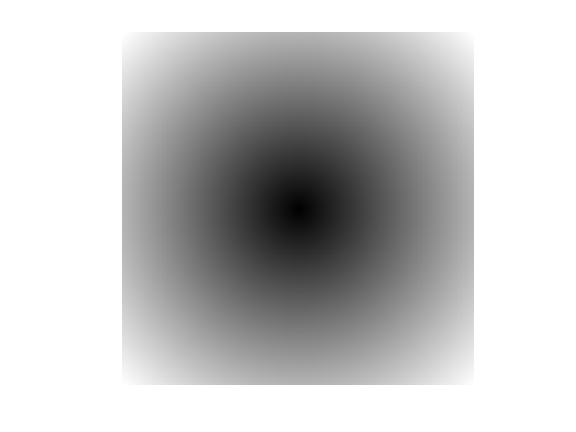Compute the texture norm. The $1/n$ normalization is intended to make the Fourier transform orthogonal.

In :
T = @(v)1/2*norm( W.*fft2(v)/n, 'fro' ).^2;
disp(['T(f) = ' num2str(T(f), 3) ] );

T(f) = 4.81e+06


The gradient of the texture norm is $$\text{Grad}T(v) = H v \quad\text{where}\quad H = F^* \text{diag}(W^2) F,$$ where $F^*$ is the inverse Fourier transform. Note that if $\eta=1$, this gradient is the inverse Laplacian $$\text{Grad}T(v) = \Delta^{-1} v.$$

Define the filtering operator $\text{Grad}T$.

In :
GradT = @(f)real(ifft2(W.^2.*fft2(f)));


This is a low pass filter.

In :
imageplot(GradT(f));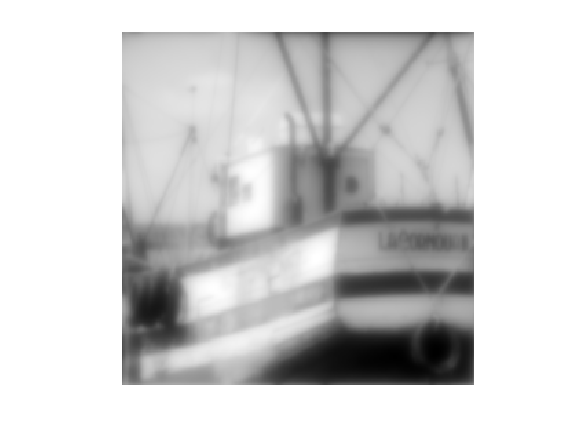Define its inverse $(\text{Grad}T)^{-1}$.

In :
GradTInv = @(f)real(ifft2(fft2(f)./W.^2));


It is a high pass filter.

In :
imageplot(GradTInv(f));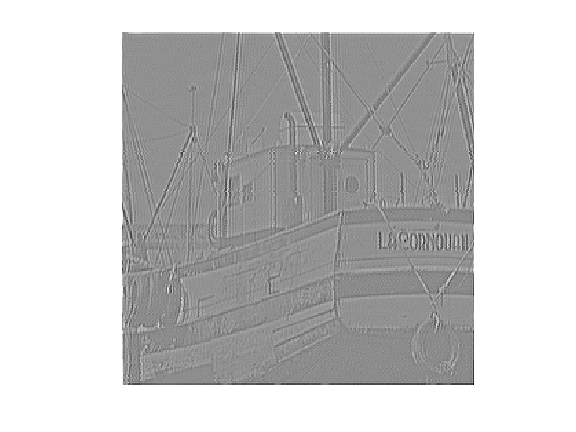## TV-$H^{-1}$ Image Decomposition¶

The TV-Hilbert decomposition solves $$\min_u \mathcal{E}(u) = \frac{1}{2} \| W F(f-u) \|^2 + \lambda J(u).$$

The mapping $u \mapsto \mathcal{E}(u)$ is a smooth functional, it can thus be minimized using a gradient descent: $$f^{(\ell+1)} = f^{(\ell)} - \tau \left( H(u-f) + \lambda \text{Grad}J(u) \right).$$

The parameter $\lambda$ for the texture/cartoon tradeoff.

In :
lambda = 5;


The gradient descent step size should satisfy: $$\tau < \frac{2}{ \max_{\omega} W_\omega^2 + \lambda \epsilon /8 }$$

In :
tau = 1.9 /( max(W(:))^2 + 8*lambda/epsilon );


Initial cartoon layer.

In :
u = f;


In :
u = u - tau * ( GradT(u-f) + lambda*GradJ(u) );


Exercise 2

Perform the gradient descent, monitor the decay of the energy.

In :
exo2()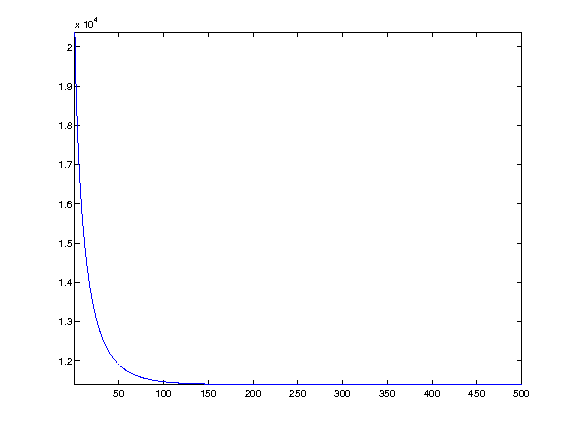In :
%% Insert your code here.


Display the cartoon layer.

In :
clf;
imageplot(u);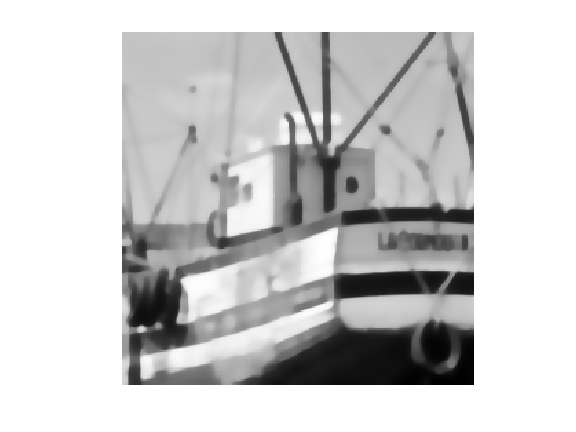Display the textured layer.

In :
clf;
imageplot( displaytexture(f-u) );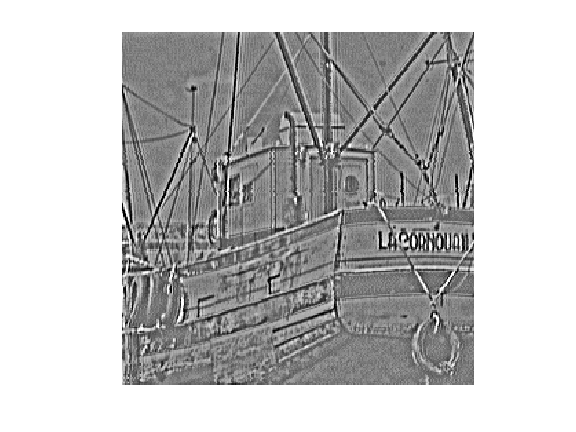## TV-Gabor Image Decomposition¶

The $H^{-1}$ texture model is intended to capture very high frequency, and thus performs poorly for medium frequency textures.

To capture these patterns, we follow:

Structure-Texture Image Decomposition - Modeling, Algorithms, and Parameter Selection, Jean-Francois Aujol, Guy Gilboa, Tony Chan, and Stanley Osher, International Journal of Computer Vision, volume 67, number 1, pages 111-136, April 2006

and we use a radial weight profile centered around a frequency $r$.

To determine the target frequency $r$, we analyse a sub-window around a point $p$ of the image containing approximately a single frequency.

Location $p$ of the sub-window.

In :
p = [125 200];


Size $\mu$, in pixels, of the sub-window.

In :
mu = 10;


In :
[Y,X] = meshgrid( 1:n, 1:n );
U = exp( ( -(X-p(1)).^2 - (Y-p(2)).^2 )/(2*mu^2)  );


In :
clf;
imageplot(U.*f);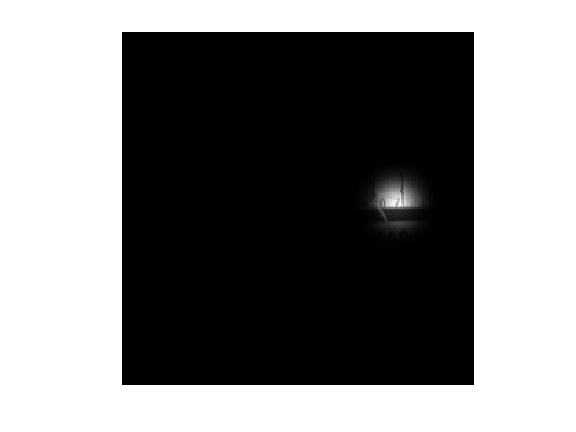Remove the low frequencies from the Fourier transform, after centering.

In :
F = fft2(U.*f);
F = fftshift(F);
F(end/2-20:end/2+20,end/2-20:end/2+20) = 0;


Compute the location $x_m,y_m$ of the pick of the spectrum.

In :
[tmp,i] = max(abs(F(:)));
[xm,ym] = ind2sub([n n], i);


Display.

In :
clf; hold on;
imageplot(abs(F));
h = plot( [ym n-ym], [xm  n-xm], 'r.' );
set(h, 'MarkerSize', 20);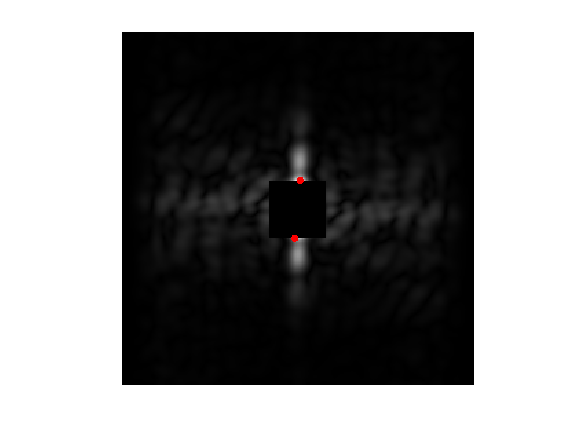Target frequency is the distance between $(x_m,y_m)$ and the center frequency.

In :
r = sqrt( (xm-n/2)^2 + (ym-n/2)^2 );


We use the following weights: $$W_\omega = 1 - e^{ -\frac{(\|\omega\|-r)^2}{ 2 \sigma^2 } }$$ where $\sigma>0$ controls the precision one expect about the frequency location.

In :
sigma = 10;
x = [0:n/2-1, -n/2:-1];
[Y,X] = meshgrid(x,x);
R = sqrt(X.^2+Y.^2);
W = 1 - exp( -(r-R).^2 / (2*sigma^2) );


Display the weight.

In :
imageplot(fftshift(W));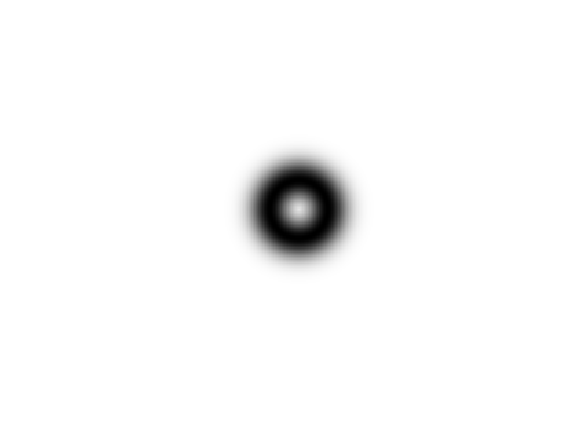Exercise 3

Define the operators $\text{Grad} T$ and apply it to an images.

In :
exo3()